﻿ Numerical Investigation of Shock Wave Decay Phenomenon in Free Atmosphere

### Numerical Investigation of Shock Wave Decay Phenomenon in Free Atmosphere

Md. Faisal Kader, M. Monjurul Ehsan, Golam Saklayen, KMR Sadi, Md. Lutfor Rahman

American Journal of Mechanical Engineering

## Numerical Investigation of Shock Wave Decay Phenomenon in Free Atmosphere

Md. Faisal Kader1,, M. Monjurul Ehsan2, Golam Saklayen1, KMR Sadi1, Md. Lutfor Rahman1

1Military Institute of Science and Technology (MIST), Mirpur Cantonment, Dhaka-1216, Bangladesh

2Islamic University of Technology (IUT), The Organization of Islamic Cooperation (OIC), Board Bazar, Gazipur-1704, Bangladesh

### Abstract

A numerical investigation of shock wave propagation in open atmosphere is presented to observe the shock wave decaying phenomena due to expansion in the free space. By examining different parameters (Pressure, velocity and density) of the upstream and downstream flow of the wave, it is possible to estimate the decaying phenomena of the wave strength. The governing equations described for compressible inviscid flow are discretized by the finite volume method. The three-dimensional Euler equations are solved by shock capturing method. Shock wave of Mach number 1.5 is allowed to flow through a shock tube and at different time intervals (35 μsec, 96 μsec, 121 μsec and 190 μsec), velocity, pressure and density variations along the center line of shock tube are investigated to observe the decay phenomenon across the shock front in open atmosphere.. The results have been compared with the Navier-Stokes Simulation (NS) results to establish the absence of viscous effects in the present flow. Due to absence of the solid boundary of the selected numerical domain in the free space, the fluid flow will act as non-viscous flow. The wave propagation in free space changes its propagation characteristics due to rapid expansion in all directions and at the same time the decay of the wave strength is observed.

• Md. Faisal Kader, M. Monjurul Ehsan, Golam Saklayen, KMR Sadi, Md. Lutfor Rahman. Numerical Investigation of Shock Wave Decay Phenomenon in Free Atmosphere. American Journal of Mechanical Engineering. Vol. 4, No. 5, 2016, pp 200-208. http://pubs.sciepub.com/ajme/4/5/5
• Kader, Md. Faisal, et al. "Numerical Investigation of Shock Wave Decay Phenomenon in Free Atmosphere." American Journal of Mechanical Engineering 4.5 (2016): 200-208.
• Kader, M. F. , Ehsan, M. M. , Saklayen, G. , Sadi, K. , & Rahman, M. L. (2016). Numerical Investigation of Shock Wave Decay Phenomenon in Free Atmosphere. American Journal of Mechanical Engineering, 4(5), 200-208.
• Kader, Md. Faisal, M. Monjurul Ehsan, Golam Saklayen, KMR Sadi, and Md. Lutfor Rahman. "Numerical Investigation of Shock Wave Decay Phenomenon in Free Atmosphere." American Journal of Mechanical Engineering 4, no. 5 (2016): 200-208.

 Import into BibTeX Import into EndNote Import into RefMan Import into RefWorks

1234
Prev Next

### 1. Introduction

The study of the shock wave propagation in the open Atmosphere is of fundamental importance in engineering applications. For designing aero-mechanism system such as transport aircraft of supersonic and hypersonic speed, the shock wave and its interaction effects with objects are the important phenomena. The shock interaction effects with objects are generally shown in aero-mechanism system and in combustion process as well as high-speed rotor flows. Many researchers investigated numerous works on shock wave propagation inside test section but very limited works on shock wave propagation in the open atmosphere were performed. Shock wave propagation in the open Atmosphere is a direct effect of the Rankine-Hugoniot relations.

Ribner [1, 2, 3] investigated that how a shock wave was perturbed by an impinging single shear wave. The theory, later on called Ribner’s theory in fact mostly based on the mode theory developed by Kovasznay  and extended to the shock problem by focusing mainly on the acoustic field generated by the interaction with the shock wave. Using his general theory of aerodynamic sound generation, Lighthill  estimated the acoustic energy scattered from the interaction of turbulence with a shock wave. Moore  analyzed the flow field produced by oblique impingement of weak plane disturbances on a normal shock wave in a reference frame fixed on the mean upstream flow. Anyiwo and Bushnell  revisited the linear analysis to identify primary mechanisms of turbulence enhancement–amplification of the vorticity mode, generation of acoustic and entropy modes from the interaction, and turbulence pumping by shock oscillations. Craig  investigated effects of various area discontinuities and perforations in a tube on the attenuation of weak shock waves. Onodera and Takayama  experimentally determined a mass flow discharge coefficient through perforated walls by considering a two-dimensional flow field. Takayama et al.  installed a porous material on the wall of a real high-speed train tunnel and found that the porous wall indeed decreased the overpressure and smeared the pressure gradient of weak shock waves generated by high-speed trains. Batley et al.  numerically studied a two-dimensional interaction process between a planar incident or reflected shock wave and a cylindrical flame based on a one-step chemical reaction. Gelfand et al. , Bartenev et al.  performed experimental and numerical investigations of deflagration and detonation initiations induced by shock focusing in a reacted medium. The effect of reflector shapes on detonation initiation was discussed. Abdellah Hadjadj and Marcello Onofri investigated the nozzle flow separation  where one-dimensional model for the numerical simulation of transport effects in small-scale shock tube is presented. The conservation equations have been integrated in the lateral directions and three-dimensional effects have been introduced as carefully controlled sources of mass, momentum and energy, into the axial conservation equations. Trimpi and Cohen  used several simplifying assumptions to suggest a practical method for taking the boundary layer effects into account for a one-dimensional approach. Wall effects in shock tubes were well described by Emrich and wheeler  and good predictions on the deviation of shock strength from the ideal condition were obtained. Mirels  presented a method to compute the attenuation of shock waves by the method of characteristics involving simplifying assumptions. Roshko  studied the effects of boundary layer growth behind the shock wave and developed a similarity solution for flow duration in shock tubes. Good agreements with the experimental results in the low pressure shock tube were achieved. Details of the laminar boundary layer in shock tubes were studied by Mirels  and some correlation formulas were proposed. Boundary layers were also experimentally observed by Chen and Emrich  to study their structure in laminar and turbulent regions of the shock tube. A numerical simulation of blast wave interaction with structure columns was conducted by Shi et al.  and their accurate estimation of blast loads on structures was essential for reliable predictions of structural response and damage. The propagation of a planar shock wave in a 90o branched duct was studied experimentally and numerically by Igra et al.  and it was shown that multiple shock wave reflections from the duct's walls caused weakening of transmitted waves and, at late times, an approach to an equilibrium, one-dimensional flow. Using a shock capturing numerical technique on the Euler equations, Rotman  numerically calculated the change in a two-dimensional turbulent flow, caused by the passage of a travelling shock wave and it was observed that the shock caused an increase in the turbulent kinetic energy.

In the present computations, three-dimensional Euler’s equation is solved by shock capturing method. HLL Reimann solver is used for shock capturing in the flow. For the better solution of the flow field, the improved three-dimensional grid adaptation technique is applied in numerical domain and the present 3D code for the solution of Euler equations has been developed by Jinnah . For the purpose of numerical solutions on shock propagation in open atmosphere, a 3D numerical code has been used. A shock tube is selected with a free space at its end wall and for the computation the 3D numerical domains are used. The time-dependent Reynolds-averaged 3D Euler equations are solved by the grid adaptation technique. All the relevant flow parameters are resolved for the shock wave of Mach number 1.5. Navier-Stokes simulation (NS) is also performed to observe the present shock wave propagation in the free space with the same incident shock Mach number.

### 2. Nomenclature

Q: Vector of conservative variables

ρ: Mass density

B: External body force

F: Inviscid flux vectors in X direction

G: Inviscid flux vectors in Y direction

H: Inviscid flux vectors in Z direction

d: Minimum dimensional length of a grid

e: Energy per unit volume

γ: Ratio of specific heats

u: Velocity in X direction

v: Velocity in Y direction

w: Velocity in Z direction

c: Characteristic velocity

P: Pressure

ρ: Density

Po: Characteristic pressure

ρ o: Characteristic density

### 3. Governing Equations

The three-dimensional Euler equations are solved by shock capturing method. Without external forces and heat sources, governing equation in three-dimensional Cartesian coordinate system is(1)

where Q = [ρ, ρu, ρv, ρw, e],

F = [ρu, ρu2, ρuv, ρuw, u(e + p)],

G = [ρv, ρuv, ρv2, ρvw, v(e + p)],

H = [ρw, ρuw, ρvw, ρw2, w(e + p)]

Here Q is the vector of conservative variables which contains mass, momentum and energy. All variables are calculated in per unit volume. Three momentum terms in three-dimensional Cartesian coordinates system are ρu, ρv and ρw per unit volume. Total energy, e is the energy terms per unit volume in these computations. F, G and H are the three inviscid flux vectors in X, Y, and Zaxis respectively. Each flux vectors contain mass flux, momentum flux and energy flux. ρu is the mass flux and ρu2, ρuv, ρuw are the momentum flux and u(e+p) is the energy flux in the X-axis. u, v and w are velocity components in each direction of Cartesian coordinates. While e is the total energy per unit volume, pressure p can be expressed by the following state equation for ideal gaswhere γ is the ratio of specific heats. The above governing equations are solved by Finite Volume Method (FVD) and the necessary formulations are given as follows: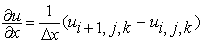(2)(3)(4)

Similarly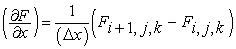(5)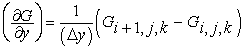(6)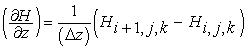(7)(8)

The Navier-stokes equations of motion for three dimensional viscous compressible fluid in Cartesian coordinates are expressed as follows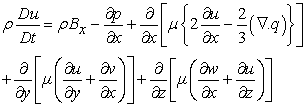(9)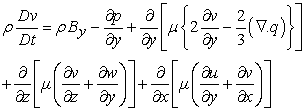(10)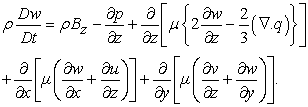(11)

### 4. Numerical Discretization and grid Adaptation

The governing equations described above for compressible inviscid flow are discretized by the finite volume method. A second order, upwind Godunov scheme of Flux vector splitting method is used to discretize the inviscid flux terms and MUSCL-Hancock scheme is used for interpolation of variables. Three dimensional hexahedral cells with adaptive grids are used for these computations. In this grid system, the cell-edge data structures are arranged in such a way that each cell contains six faces which are sequence in one to six and each face indicates two neighboring cells that are left cell and right cell providing all faces of a cell are arranged in a particular way by positions and coordinates in the grid system.Download asVeiw figureFigures index
Figure 1. (i) Three-dimensional cell with six faces; (ii) Each face addresses the center of two neighboring cells, i and j

The grid adaptation is one of the improved and computational time saving techniques, which is used in these computations. The grid adaptation is performed by two procedures, one is refinement procedure and another is coarsening procedure. The refinement and coarsening operations are handled separately in computation. The level of refinement, used in all types of computation, is 2 or 3, depending on the number of cell per unit volume. For the original grid size of 5x5x5 (mm), the refined grid size will be 1.25x1.25x1.25 (mm) if refinement level is 2. Similarly for the original grid size of 10x10x10 (mm), the refined grid size will be 1.25x1.25x1.25 (mm) if refinement level is 3. So it is easy to perform the grid convergence test by using different grid sizes and different refinement levels. The three-dimensional grid adaptation technique for grid size 5x5x5 (mm) is shown in Figure 2 which is the sectional view of half numerical domain. Similarly for grid size 10x10x10 (mm) is shown in Figure 3 which is the sectional view of half numerical domain.Download asVeiw figureFigures index
Figure 2. Sectional view of half numerical domain of grid size 5x5x5 (mm) where 3D grid adaptation technique is shownDownload asVeiw figureFigures index
Figure 3. Sectional view of half numerical domain of grid size 10x10x10 (mm) where 3D grid adaptation technique is shown

### 5. Numerical Setup and Boundary Condition

The grid size as shown in Figure 4, before adaptation is 5 x 5 x 5 (mm) and the initial number of grid is 10240. Similarly the grid size as shown in Figure 5, before adaptation is 10 x 10 x 10 (mm) and the initial number of grid is 1280. Both numerical domains are used for grid convergence study. Each domain has two sections, one is the shock tube and another is the free space at the end of the shock tube. The dimensions of width and depth of shock tube is 40x40 (mm). The selected length of the shock tube in the domain is 80 mm and at the end of this shock tube a free space region of 80 x 120 x 120 (mm) is taken for the shock wave propagation in the Open Atmosphere. The longitudinal distances (x/d) of any point on the centerline of the shock tube are determined from the starting point of the shock tube where d is the minimum dimensional length of a grid in the grid structure before adaptation and it is taken as d = 5 mm.The value x/d = 0 is the end point of the shock tube and the region between x/ d = 0 and x/d = 16 is used as the free space region. All parameters (velocity, pressure and density) are computed along the center line of the shock tube. In these computations, the threshold values for refinement are used 0.24~0.28 and the threshold values for coarsening are used 0.20~0.24 and the level of refinement is 2 and 3. Based on characteristic values and shock wave mach number of 1.5, Reynolds number is 21546 and Prandtl number is 0.722.Download asVeiw figureFigures index
Figure 4. 3D numerical domain where the grid size is 5x5x5 (mm)Download asVeiw figureFigures index
Figure 5. 3D numerical domain where the grid size is 10x10x10 (mm)

### 6. Grid Convergence Study

The study of the grid convergence on the present computational results has been carried out for different levels of grid refinement and for different grid sizes. The refinement level two is used to solve the flow field with coarse grid and the refinement level three is used to solve the flow field with very refined grid. The results, obtained for level two and level three refined grids are compared to observe the accuracy level of the computational results and such comparisons are used to determine the convergence behavior of the present simulation results. It is observed that the solutions of shock wave in open atmosphere for different refinement levels and for different grid sizes have the good agreement.

### 7. Data Processing

The purpose of the present work to provide a comprehensive and accurate description of shock wave propagation in the open atmosphere. The shock wave of Mach number 1.5 is allowed to propagate from the end section of the shock tube. 80x120x120 (mm) domain is used at the end section of the shock tube to generate the numerical grids. All wall boundaries of the selected domain are considered as open boundaries to make it as equivalent to free space. The Navier-Stokes simulation (NS) results are used to compare with the present simulation results and it is observed that there have good agreements between the present simulation results and the NS results. To measure the different parameters of the upstream and downstream flow of the wave, 22 points of equal spacing are selected along the center line of the shock tube, as shown in Figure 6, in them, 8 points are selected inside the shock tube which are used to get the incident wave information and the rest of 14 points are selected outside of the end section of the shock tube which are used to establish the decaying phenomena of the wave propagation in the free space. All flow parameters (velocity, pressure and density) are computed on these 22 points and the measured values indicate the wave characteristics values along the longitudinal direction (wave propagation direction, x-axis).Download asVeiw figureFigures index
Figure 6. Schematic layout of the shock tube and the relative position of the free space

### 8. Results and Discussion

For the shock wave position as shown in Figure 7(i) which is just near the end section in the free space, the longitudinal velocity, U/c variations are determined along the center line using different grid sizes of 5x5x5 (mm) and 10x10x10 (mm). c is the characteristic velocity. Figure 8(i) shows the longitudinal velocity profiles across the shock wave when the shock wave just starts to travel in the free space and the wave travelled time is t = 35 μsec and it is observed that the decay of the wave strength increases with an increase of travelled time in the free space. Also it is observed that the velocity obtained using different grid sizes satisfies the grid convergence study. Navier-Stokes equations are also solved to compute the longitudinal velocity variation along the center line in the free space with the same incident shock Mach number. The comparisons between the Navier-Stokes simulation (NS) results and the present simulation results on velocity are performed and it is observed that there have good agreements. Similarly Figure 8(ii), (iii) and (iv) show the longitudinal velocity profiles for the wave propagating time 96 μsec, 121 μsec and 190 μsec respectively in the free space, where the position of the wave is shown in Figure 7(ii), (iii) and (iv) and it is observed that the shock induced longitudinal flow velocity decreases in all cases for the different wave positions which indicates the decay of the wave strength. Also the longitudinal velocity from NS results for the travelled time 35 μsec, 96 μsec, 121 μsec and 190 μsec of the wave have the good agreements with the present simulation results. Form Figure 8 it is evident that due rapid expansion and decay of shock strength, around the end of shock tube velocity is increased with wave travelled time.Download asVeiw figureFigures index
Figure 7. Shock wave position from the end section of the shock tube in the free space after the wave travelled time (i) t = 35 μsec, (ii) t = 96 μsec, (iii) t = 121 μsec, (iv) t = 190 μsec

The normalized pressure, P/Po variations are determined along the center line using different grid sizes of 5x5x5 (mm) and 10x10x10 (mm). Po is the characteristic pressure, taken as Atmospheric pressure. Figure 9(i) shows the normalized pressure profiles across the shock wave when the shock wave just starts to travel in the free space and the wave travelled time, t = 35 μsec and it is observed that the wave strength decreases with the increase of the travelled time in the free space. Also it is observed that the pressure obtained using different grid sizes satisfies the grid convergence study. With an increase of wave travelling time, there is a fall of pressure near the end of shock tube which is similar to an expansion wave behind the shock tube. Similarly the normalized density, ρ/ρ o variations are determined along the center line using different grid sizes of 5x5x5 (mm) and 10x10x10 (mm). ρ o is the characteristic density. Figure 10(i) shows the normalized density profiles across the shock wave when the shock wave travelled time, t = 35 μsec and it is observed that the decay of the wave strength increases with an increase of the travelled time in the free space. Figure 10(ii), (iii) and (iv) show the normalized density profiles for the wave propagating time 96 μsec, 121 μsec and 190 μsec respectively in the free space, where the position of the wave is shown in Figure 7(ii), (iii) and (iv) and it is observed that the normalized density decreases across the wave in all cases for the different wave positions which indicates the decay of the wave strength. Also the normalized density from NS results for the travelled time 35 μsec, 96 μsec, 121 μsec and 190 μsec of the wave have the good agreements with the present simulation results. Due to the creation of expansion wave, as the shock front moves forward with time, the density around the end of shock tube is decreased.Download asVeiw figureFigures index
Figure 8. Longitudinal velocity, U/c profiles along the centerline of the free space using different grid sizes when the wave travelled time; (i) t = 35 μsec, (ii) t = 96 μsec, (iii) t = 121 μsec, (iv) t = 190 μsecDownload asVeiw figureFigures index
Figure 9. Normalized pressure, P/Po profiles along the centerline of the free space using different grid sizes when the wave travelled time; (i) t = 35 μsec, (ii) t = 96 μsec, (iii) t = 121 μsec, (iv) t = 190 μsecDownload asVeiw figureFigures index
Figure 10. Normalized density, ρ/ρo profiles along the centerline of the free space using different grid sizes when the wave travelled time; (i) t = 35 μsec, (ii) t = 96 μsec, (iii) t = 121 μsec, (iv) t = 190 μsecDownload asVeiw figureFigures index
Figure 11. Lateral velocity, v/c profiles along the centerline of the free space using different grid sizes when the wave travelled time; (i) t = 35 μsec, (ii) t = 96 μsec, (iii) t = 121 μsec, (iv) t = 190 μsecDownload asVeiw figureFigures index
Figure 12. Lateral velocity, w/c profiles along the centerline of the free space using different grid sizes when the wave travelled time; (i) t = 35 μsec, (ii) t = 96 μsec, (iii) t = 121 μsec, (iv) t = 190 μsec

The lateral velocities v/c and w/c profiles across the shock wave are shown in Figure 11 and Figure 12 respectively. In the present computation, the longitudinal velocity along the x-direction is the normal velocity on yz-plane and because of the zero velocity on other y and z direction, the tangential velocity of the wave in the free space is zero. So the wave is always propagating in the normal direction. Similarly if the wave propagates in y-axis, that is, the direction is normal on zx-plane, then the tangential velocity along x and z axis will be zero. Here the shock wave is allowed to propagate in X direction which is considered as normal direction, so the velocities at y and z directions are the tangential components which are zero because the wave front is spherical in shape in open atmosphere. So the propagation is always observed in radial direction and the surfaces of the wave propagation in the open Atmosphere have the tendency to be spherical in shape.

### 9. Conclusion

The shock wave propagation in the open atmosphere has been investigated by means of Euler simulation where the flow is considered as non-viscous flow. The results have been compared with the Navier-Stokes Simulation (NS) results to establish the absence of viscous effects in the present flow. Due to absence of the solid boundary of the selected numerical domain in the free space, the fluid flow acts as a non-viscous flow. To establish the decay phenomenon of shock strength, several points are chosen along the centerline of shock tube to obtain the information of the change of parameters (pressure, velocity and density) inside the shock tube as well as in outside the shock tube in open atmosphere. For different wave travelling time intervals (35 μsec, 96 μsec, 121 μsec and 190 μsec) it has been observed that with an increase of wave travelled time, wave strength is decayed and around the end of shock tube an increase of velocity is observed due to the rapid expansion when the wave exposed to open atmosphere. Moreover, negative pressure and density formation is found near the end of shock tube due to the formation of expansion wave as the shock wave moves in forward direction. In the present study the spherical expansion is observed as well by numerical imaging. It is observed that the computational results are completely independent on the grid size. The 3D numerical solutions have the advantages to know properly the expansion phenomena of the wave in space and the 3D numerical visualization indicates that the wave front during propagation in open atmosphere is always spherical in shape. Numerical techniques for such types of propagation are more suitable to get the reliable results and easily estimate the physical data structure which is difficult to measure in experiment.

### References

  Ribner, H. S. 1953, “Convection of a pattern of vorticity through a shock wave.” NACA TN-2864, 1953.In article  Ribner, H. S. 1954, “Shock-turbulence interaction and the generation of noise.” NACA TN-3255, 1954.In article  Ribner, H. S. 1969, “Acoustic energy flux from shock-turbulence interaction.” Journal of Fluid Mechanics Vol. 35, 1969, pp. 299-310.In article View Article  Kovasznay, L. S. G. 1953, “Turbulence in supersonic flow.” J. Aero. Sci. Vol. 20, pp. 657-682.In article  Lighthill, M. J. 1953, “On the energy scattered from the interaction of turbulence with sound or shock wave.” Proc. Camb. Phil. Soc. 49, 531-551.In article View Article  Moore, F. K. 1953, “Unsteady oblique interaction of a shock wave with a plane disturbances.” NACA TN-2879, 1953.In article  Anyiwo, J. C. & Bushnell, D. M. 1982, “Turbulence amplification in shock-wave boundary-layer interaction.” AIAA J. Vol. 20, 893-899.In article View Article  Craig JE, 1977, “Shock waves in open ended ducts with complex geometry.” Ph D Aeronautics, California Institute of Technology, Pasdene CA, USA.In article  Onodera H, Takayama K, 1992, “An analysis of shock wave propagation over perforated wall and its discharge coefficient”, Trans Jpn Soc Mech Eng B (in Japanese). 58:1408.In article View Article  Takayama K, Sasoh A, Onodera O, Kaneko R, Matsui Y, 1995, “Experimental investigation on tunnel sonic boom”. Shock Waves 5:127(138).In article  Batley, G.A., McIntosh, A.C., Brindley, J., Falle, S.A.E.G., 1994, “A numerical study of the vorticity field generated by the baroclinic effect due to the propagation of a planar pressure wave through a cylindrical premixed laminar flame”. J. Fluid Mech. 279, 217-237.In article View Article  Gelfand, B.E., Khomik, S.V., Bartenev, A.M., Medvedev, S.P., Groing, H., Olivier, H., 2000, “Detonation and deflagration at the focusing of shockwaves in combustible gaseous mixture” Shock Waves, 10, 197-204.In article View Article  Bartenev, A.M., Khomik, S.V., Gelfand, B.E., Gronig, H., Olivier, H., 2000, “Effect of reflection type on detonation initiation at shock-wave focusing.” Shock Waves 10, 205-215.In article View Article  Abdellah Hadjadj, Marcello Onofri, 2009, “Nozzle flow separation”. Shock Waves, 19:163-169.In article View Article  Trimpi, R.L., Cohen, N.B., 1955, “A theory for predicting the flow of real gases in shock tubes with experimental verification.” Technical Report NACA 3375, National Advisory Committee for Aeronautics.In article  Emrich, R.J., Wheeler, D.B., 1958, “Wall effects in shock tube flow” Phys. Fluids 1, 14-23.In article View Article  Mirels, H., 1957, “Attenuation in a shock tube due to unsteady-boundary layer action.” Technical Report TN 1333, National Advisory Committee for Aeronautics.In article  Roshko, A., 1960, “On flow duration in low-pressure shock tubes.” Phys. Fluids 3(6), 835-842.In article View Article  Mirels, H., 1966, “Correlation fomulas for laminar shock tube boundary layer.” Phys. Fluids 9(7), 1265-1272.In article View Article  Chen, C.J., Emrich, R.J., 1963, “Ivestigation of the shock-tube boundary layer by a tracer method.”, Phys. Fluids 6(1), 1-9.In article View Article  Yanchao Shi, Hong Hao and Zhong-Xian Li, 2007, “Numerical simulation of blast wave interaction with structure columns.”, Shock Waves Journal, Vol. 17: 113-33.In article View Article  O. Igra, L. Wang, J. Falcovitz and W. Heilig, 1998, “Shock wave propagation in a branched duct.” Shock Waves Journal, Vol. 8: 375-81.In article View Article  Rotman D, 1991, “Shock wave effects on a turbulent flows.” Phys. Fluids A3: 1792-806.In article View Article  Jinnah MA, 2005, “Numerical and experimental study of Shock/Turbulent flow interaction.” Doctor Thesis, Tohoku University.In article